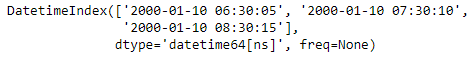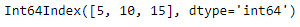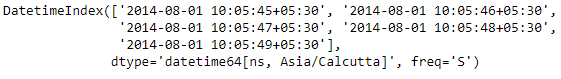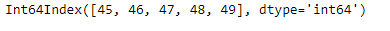# Python | Pandas DatetimeIndex.second

Python is a great language for doing data analysis, primarily because of the fantastic ecosystem of data-centric python packages. Pandas is one of those packages and makes importing and analyzing data much easier.

Pandas` DatetimeIndex.second` attribute outputs an Index object containing the second values present in each of the entries of the DatetimeIndex object.

Syntax: DatetimeIndex.second

Return: Index containing seconds.

Example #1: Use `DatetimeIndex.second` attribute to find the seconds value present in the DatetimeIndex object.

 `# importing pandas as pd ` `import` `pandas as pd ` ` `  `# Create the DatetimeIndex ` `didx ``=` `pd.DatetimeIndex([``'2000-01-10 06:30:05'``, ``'2000-01-10 07:30:10'``, ` `                                                ``'2000-01-10 08:30:15'``]) ` ` `  `# Print the DatetimeIndex ` `print``(didx) `

Output :Now we want to find all the seconds value present in the DatetimeIndex object.

 `
` `# find all the second values present in the object ` `didx.second `

Output :As we can see in the output, the function has returned an Index object containing the second values present in each entry of the DatetimeIndex object. Here 5, 10 and 15 are the second values in the entries of the DatetimeIndex object.

Example #2: Use `DatetimeIndex.second` attribute to find the seconds value present in the DatetimeIndex object.

 `# importing pandas as pd ` `import` `pandas as pd ` ` `  `# Create the DatetimeIndex ` `# Here 'S' represents secondly frequency  ` `didx ``=` `pd.DatetimeIndex(start ``=``'2014-08-01 10:05:45'``, freq ``=``'S'``,  ` `                              ``periods ``=` `5``, tz ``=``'Asia/Calcutta'``) ` ` `  `# Print the DatetimeIndex ` `print``(didx) `

Output :Now we want to find all the seconds value present in the DatetimeIndex object.

 `# find all the second values present in the object ` `didx.second `

Output :As we can see in the output, the function has returned an Index object containing the second values present in each entry of the DatetimeIndex object.

My Personal Notes arrow_drop_upCheck out this Author's contributed articles.

If you like GeeksforGeeks and would like to contribute, you can also write an article using contribute.geeksforgeeks.org or mail your article to contribute@geeksforgeeks.org. See your article appearing on the GeeksforGeeks main page and help other Geeks.

Please Improve this article if you find anything incorrect by clicking on the "Improve Article" button below.

Article Tags :

1

Please write to us at contribute@geeksforgeeks.org to report any issue with the above content.